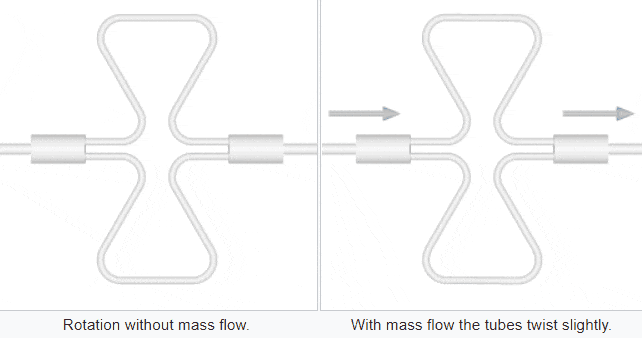# Animation of Coriolis Meter

Coriolis Meter is a Direct type Flow Measurement instrument and Measures the Mass Flow. When there is no flow through the tubes then the tubes vibrate at a resonant frequency.

When there is a flow through the tubes then depends on the flow & its mass the tubes vibration will change. The change in vibration is measured and it is proportional to Mass Flow.

## Coriolis Meter

Video Courtesy : Khrone

A mass flow meter, also known as an inertial flow meter is a device that measures mass flow rate of a fluid traveling through a tube. The mass flow rate is the mass of the fluid traveling past a fixed point per unit time.

The mass flow meter does not measure the volume per unit time (e.g., cubic meters per second) passing through the device; it measures the mass per unit time (e.g., kilograms per second) flowing through the device.

Volumetric flow rate is the mass flow rate divided by the fluid density. If the density is constant, then the relationship is simple. If the fluid has varying density, then the relationship is not simple. The density of the fluid may change with temperature, pressure, or composition, for example.

The fluid may also be a combination of phases such as a fluid with entrained bubbles. Actual density can be determined due to dependency of sound velocity on the controlled liquid concentration.

There are two basic configurations of coriolis flow meter: the curved tube flow meter and the straight tube flow meter. This article discusses the curved tube design.

## Straight Tube Coriolis Flow Meter

The animations on the right do not represent an actually existing Coriolis flow meter design. The purpose of the animations is to illustrate the operating principle, and to show the connection with rotation.Fluid is being pumped through the mass flow meter. When there is mass flow, the tube twists slightly. The arm through which fluid flows away from the axis of rotation must exert a force on the fluid, to increase its angular momentum, so it bends backwards.

The arm through which fluid is pushed back to the axis of rotation must exert a force on the fluid to decrease the fluid’s angular momentum again, hence that arm will bend forward.

In other words, the inlet arm (containing an outwards directed flow), is lagging behind the overall rotation, the part which in rest is parallel to the axis is now skewed, and the outlet arm (containing an inwards directed flow) leads the overall rotation.

## Curved Tube Coriolis Flow Meter

The animation on the right represents how curved tube mass flow meters are designed. The fluid is led through two parallel tubes. An actuator (not shown) induces equal counter vibrations on the sections parallel to the axis, to make the measuring device less sensitive to outside vibrations.

The actual frequency of the vibration depends on the size of the mass flow meter, and ranges from 80 to 1000 Hz. The amplitude of the vibration is too small to be seen, but it can be felt by touch.

When no fluid is flowing, the motion of the two tubes is symmetrical, as shown in the left animation. The animation on the right illustrates what happens during mass flow: some twisting of the tubes.Left Fig : Coriolis Mass Flow Meter with No Flow

The arm carrying the flow away from the axis of rotation must exert a force on the fluid to accelerate the flowing mass to the vibrating speed of the tubes at the outside (increase of absolute angular momentum), so it is lagging behind the overall vibration.

The arm through which fluid is pushed back towards the axis of movement must exert a force on the fluid to decrease the fluid’s absolute angular speed (angular momentum) again, hence that arm leads the overall vibration.Right Fig : Coriolis Mass Flow Meter with Flow

The inlet arm and the outlet arm vibrate with the same frequency as the overall vibration, but when there is mass flow the two vibrations are out of sync: the inlet arm is behind, the outlet arm is ahead. The two vibrations are shifted in phase with respect to each other, and the degree of phase-shift is a measure for the amount of mass that is flowing through the tubes.

#### Articles You May Like :

Flow Control Loop Controller

In Line Flow Switch Operation

What is Transit Time Meter ?

Differential Pressure Flow meter

Orifice vs Venturi Flow Meter

Don't Miss Our Updates
Be the first to get exclusive content straight to your email.
We promise not to spam you. You can unsubscribe at any time.

### 3 thoughts on “Animation of Coriolis Meter”

1.I had lot of confusion regarding Coriolis flow meter working principle . With your demonstration and animation it is more or less clear. Thank you.

2.3.